Hi Mike. I had a question about question 10 on page 218 from your PWN 4th edition Math guide book. If percent means divide by 100, why is it 8 x10^-4 instead of 8 x 10^-8? I thought I had to divide 8 x 10^-6 by a 100. Could you clear this up for me?

Sure. I think what you’re missing here is that the percent signs are in the answer choices, so you have to divide the various choices by 100, not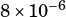. But let’s take a step back.

The calculation we’re doing here is dividing about 2600 by 309.3 million. When I do that I get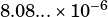. Expanding that from scientific notation I have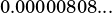So we’re looking for the answer choice that matches that.

The percent sign does mean divide by 100 (or move the decimal point two places to the left). Let’s convert choice B,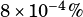, in two steps.

First, apply the scientific notation, so we have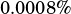.

Now apply the % sign by dividing by 100 (or moving the decimal point two places to the left). Now we have, which matches that we wanted.

Does that help?